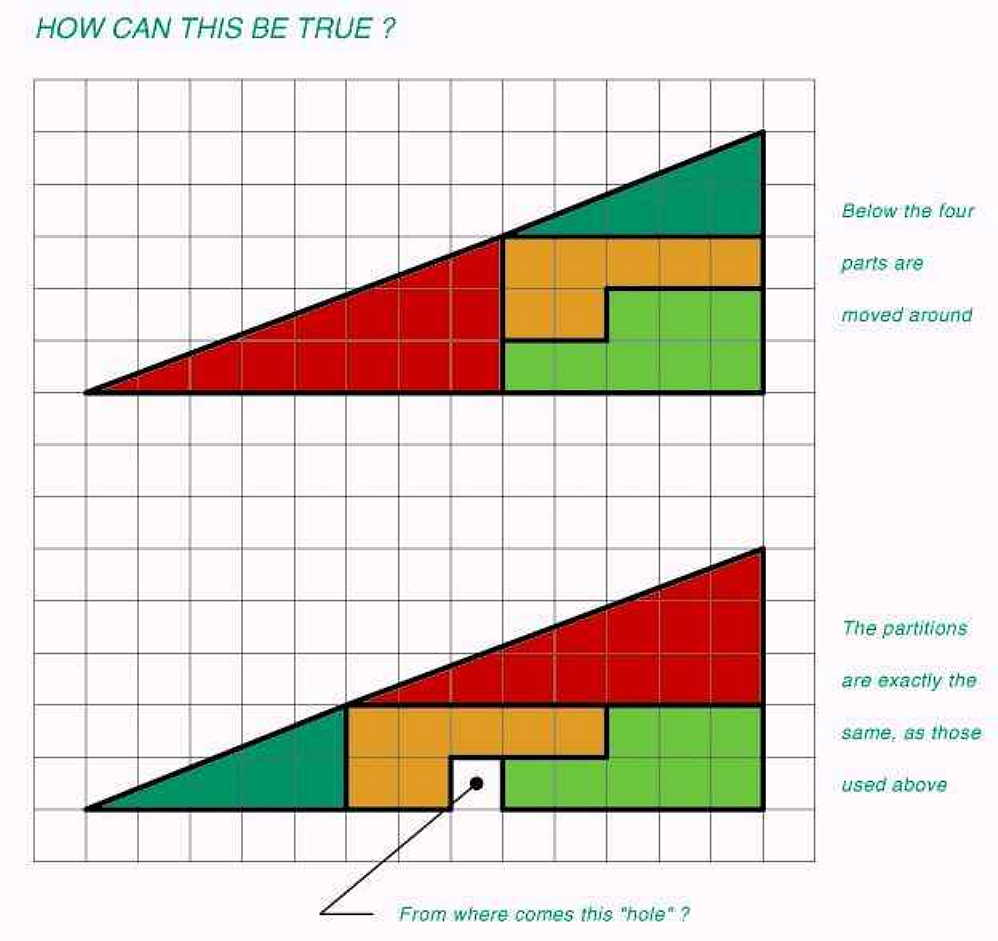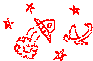## Apparent variable area of a triangle by VK3UKF 2006.

##### Please do not make comment on this without having tried it out first. Unless you're sure !

About 2 years ago, (2004) an aquaintance passed only the image on this page, on to me. No explanation.

----------------------------------------------------------------

After you have made the appropriate shapes out of either paper (from printing the image out), or plywood or hardboard that fits into a recessed cutout of the same dimensions. (Like a child's jigsaw puzzle that has 4 pieces.)
You will, no doubt, feel exhilaration as you discover that you can actually do it, and it works repeatedly.
You will want to run and show your friends this new discovery.
Then you will try to figure it out.
You will fail.
You will then go slightly crazy or mad.
You will then feel pain as logic escapes you totally.

I will save you.

The answer is to actually show it to your friends, and watch them as they experience and display exactly what you just did.

----------------------------------------------------------------Using the dimensions shown in the image above, the area of a triangle is apparently variable.
The possibilities as to the utilisation of this information boggle the imagination.
Real-estate sub-divisions tend to come to mind straight away.

To save the image, right click, then click 'save target as'. File size 72 kb.

----------------------------------------------------------------
IT JUST DOESN'T ADD UP !
----------------------------------------------------------------

THE MAIN TRIANGLE

The base is 13 units in length.
The vertical side forming the right angle with the base is 5 units in height.
The length of the hypotenuse opposite the right angle is the sqr root of (13 sqrd (169) + 5 sqrd (25) = 194) = 13.92838828 units. The angles either side of the hypotenuse are
Tangent of theta is 5/13 = 0.384615384. Arctangent of 0.384615384 is 21.037511 degrees,
therefore the other angle is 90 - 21.037511 = 68.962489 degrees.

THE SMALL GREEN TRIANGLE

The base is 5 units in length.
The vertical side forming the right angle with the base is 2 units in height.
The length of the hypotenuse opposite the right angle is the sqr root of (5 sqrd (25) + 2 sqrd (4) = 29) = 5.385164807 units.
The angles either side of the hypotenuse are
Tangent of theta is 2/5 = 0.4. Arctangent of 0.4 is 21.80140949 degrees,
therefore the other angle is 90 - 21.80140949 = 68.19859051 degrees.

THE LARGE RED TRIANGLE

The base is 8 units in length.
The vertical side forming the right angle with the base is 3 units in height.
The length of the hypotenuse opposite the right angle is the sqr root of (8 sqrd (64) + 3 sqrd (9) = 73) = 8.544003745 units.
The angles either side of the hypotenuse are
Tangent of theta is 3/8 = 0.375. Arctangent of 0.375 is 20.55604522 degrees,
therefore the other angle is 90 - 20.55604522 = 69.44395478 degrees.

WHAT ???? Am I doing wrong???, please let me know via email.
If you make a lot of money from this, please don't forget me.

Thank you Sterling, I couldn't see the trees for the forest. :-)

-----------------------------------------------------------------------------------------------------------------------------

As explanations and comments arrive, I will place them here.

Answers supplied by Sterling K. Webb. March 2006

http://mathforum.org/library/drmath/view/61087.html

http://www.nobeliefs.com/puzzles/triangle-puzzle.htm
(They want you to "contribute" before they tell you the answer...)

http://www.puzzle.dse.nl/harder/index_us.html#appearing_area

And here's the website your "friend" got the picture from, only he didn't give you the answer, which is on the same website: